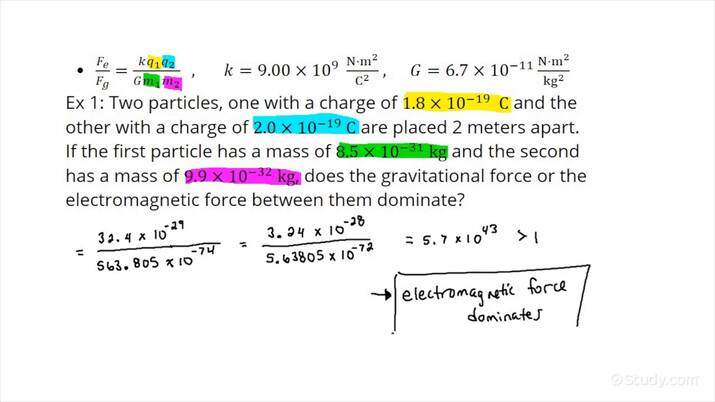# How to Determine Whether Gravitational or Electromagnetic Forces Dominate Between 2 Objects of Known Charge & MassAn error occurred trying to load this video.

Try refreshing the page, or contact customer support.

• 0:04 Introduction
• 0:37 Example 1 -…
• 4:31 Example 2 -…
Daniel Jibson, Kathryn Boddie
• Instructors
Daniel Jibson

Daniel has taught physics and engineering since 2011. He has a BS in physics-astronomy from Brigham Young University and an MA in science education from Boston University. He currently holds a science teaching license for grades 8-12.

•
Kathryn Boddie

Kathryn has taught high school or university mathematics for over 10 years. She has a Ph.D. in Applied Mathematics from the University of Wisconsin-Milwaukee, an M.S. in Mathematics from Florida State University, and a B.S. in Mathematics from the University of Wisconsin-Madison.

## How to Determine Whether Gravitational or Electromagnetic Forces Dominate Between 2 Objects of Known Charge & Mass

Step 1: Make a list of the known charges and masses of each object.

Step 2: Take a ratio of the electric force between the objects to the gravitational force between the objects: {eq}\dfrac{F_e}{F_g} = \dfrac{kq_1q_2}{Gm_1m_2} {/eq}.

Step 3: Declare the electric force to be dominant if the absolute value of the ratio of electric force to gravitational force is much larger than one. The gravitational force dominates if the absolute value of the ratio is much smaller than one.

## What are Gravitational and Electric Forces?

Gravitational Force: The gravitational force between two objects depends upon the mass of each object, {eq}m_1 {/eq} and {eq}m_2 {/eq}, and the distance, {eq}r {/eq}, separating the centers of the objects. The equation relating the gravitational force, {eq}F_g {/eq}, and these quantities is:

$$F_g = \dfrac{Gm_1m_2}{r^2}$$

In this equation, {eq}G {/eq} is the universal gravitational constant which has a value of {eq}6.7 \times 10^{-11}\ \frac{\rm{N}\cdot \rm{m^2}}{kg^2} {/eq}.

Electric Forces: Two objects with net charges {eq}q_1 {/eq} and {eq}q_2 {/eq} exert a force upon each other whose magnitude is dependent upon their charges and the distance, {eq}r {/eq}, separating them. The equation is similarly structured to the gravitational force equation:

$$F_e = \dfrac{kq_2q_2}{r^2}$$

In this equation, the constant {eq}k {/eq} is known as the Coulomb constant and has a value of {eq}9.00 \times 10^{9}\ \frac{N\cdot m^2}{C^2} {/eq}.

Taking the ratio of these two forces, we get:

$$\dfrac{F_e}{F_g} = \dfrac{\dfrac{kq_1q_2}{r^2}}{\dfrac{Gm_1m_2}{r^2}}$$

Since the distance of separation between the objects is the same for both electric and gravitational forces, the {eq}r^2 {/eq} term cancels and the equation becomes:

$$\dfrac{F_e}{F_g} = \dfrac{kq_1q_2}{Gm_1m_2}$$

The following two problems demonstrate how to determine whether gravitation or electromagnetic forces dominate between two objects given their charges, masses, and separation.

## Examples of Determining Whether Gravitational or Electromagnetic Forces Dominate Between 2 Objects of Known Charge & Mass

#### Example 1

Two electrons, each with a charge of {eq}1.6 \times 10^{-19}\ \rm{C} {/eq} and a mass of {eq}9.1 \times 10^{-31}\ \rm{kg} {/eq} are placed 1 meter apart. Does the gravitational or electric force between them dominate in this case?

Step 1: Make a list of the known charges and masses of each object.

We know the following information:

• {eq}m_1 = m_2 = 9.1 \times 10^{-31}\ \rm{kg} {/eq}
• {eq}q_1 = q_2 = 1.6 \times 10^{-19}\ \rm{C} {/eq}

Step 2: Take a ratio of the electric force between the objects to the gravitational force between the objects: {eq}\dfrac{F_e}{F_g} = \dfrac{kq_1q_2}{Gm_1m_2} {/eq}.

The ratio for these two electrons is:

$$\dfrac{F_e}{F_g} = \dfrac{\left ( 9.00 \times 10^{9}\ \frac{N\cdot m^2}{C^2} \right )(1.6 \times 10^{-19}\ \rm{C})(1.6 \times 10^{-19}\ \rm{C})}{\left ( 6.7 \times 10^{-11}\ \frac{\rm{N}\cdot \rm{m^2}}{kg^2} \right )(9.1 \times 10^{-31}\ \rm{kg})(9.1 \times 10^{-31}\ \rm{kg})} \approx 4.2 \times 10^{42}$$

Step 3: Declare the electric force to be dominant if the absolute value of the ratio of electric force to gravitational force is much larger than one. The gravitational force dominates if the absolute value of the ratio is much smaller than one.

In this case, the electric force is 42 orders of magnitude greater than the gravitational force between the electrons. In effect, the gravitational force is negligible and the electric force dominates.

#### Example 2

Two large, metal, conducting spheres, with masses of 1000 kg and 500 kg are given a net charge. The lighter sphere has a charge of {eq}3.5 \times 10^{-9}\ \rm{C} {/eq} and the heavier sphere has a charge of {eq}8.4 \times 10^{-9}\ \rm{.C} {/eq}. Does the gravitational or electric force between these two spheres dominate in this case?

Step 1: Make a list of the known charges and masses of each object.

We know the following information:

• {eq}m_1 = 1000\ \rm{kg} {/eq}
• {eq}m_2 = 500\ \rm{kg} {/eq}
• {eq}q_1 = 8.4 \times 10^{-9}\ \rm{C} {/eq}
• {eq}q_2 = 3.5 \times 10^{-9}\ \rm{C} {/eq}

Step 2: Take a ratio of the electric force between the objects to the gravitational force between the objects: {eq}\dfrac{F_e}{F_g} = \dfrac{kq_1q_2}{Gm_1m_2} {/eq}.

The ratio of the forces is:

$$\dfrac{\left ( 9.00 \times 10^{9}\ \frac{N\cdot m^2}{C^2} \right )(3.5 \times 10^{-9}\ \rm{C})(8.4 \times 10^{-9}\ \rm{C})}{\left ( 6.7 \times 10^{-11}\ \frac{\rm{N}\cdot \rm{m^2}}{kg^2} \right )(1000\ \rm{kg})(500\ \rm{kg})} \approx 7.9 \times 10^{-3}$$

Step 3: Declare the electric force to be dominant if the absolute value of the ratio of electric force to gravitational force is much larger than one. The gravitational force dominates if the absolute value of the ratio is much smaller than one.

In this case, the gravitational force dominates, being about 1000 times stronger than the electric force.